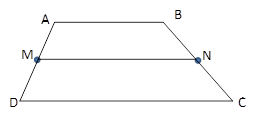Chapter 4.4, Problem 16EElementary Geometry For College St...

7th Edition
Alexander + 2 others
ISBN: 9781337614085

Solutions

Chapter
SectionElementary Geometry For College St...

7th Edition
Alexander + 2 others
ISBN: 9781337614085
Textbook Problem

In Exercises 11 to 16, the drawing shows trapezoid ABCD with A B ¯ | | D C ¯ ; also, M and N are midpoints of A D ¯ and B C ¯ , respectively.Exercises 11-16Given: A B = x + 3 y + 4 and D C = 3 x + 5 y − 2 .Find: MN, in terms of x         a n d         y .

To determine

To Find:

MN in terms of xandy for the trapezoid ABCD.

Explanation

Consider the following trapezoid ABCD.

Given:

AB=x+3y+4 and DC=3x+5y2.

Also in the given trapezoid, AB¯||DC¯.

M is the midpoint of the side AD¯ and N is the midpoint of the side BC¯.

MN is the median of the trapezoid ABCD

Still sussing out bartleby?

Check out a sample textbook solution.

See a sample solution

The Solution to Your Study Problems

Bartleby provides explanations to thousands of textbook problems written by our experts, many with advanced degrees!

Get Started

Fill in each blank: 3lb5oz=oz

Elementary Technical Mathematics

53-54 Find f(n)(x). f(x)=2x

Calculus (MindTap Course List)

True or False: div curl F = 0.

Study Guide for Stewart's Multivariable Calculus, 8th

True or False: dmdv stands for the derivative of the function m with respect to the variable v.

Study Guide for Stewart's Single Variable Calculus: Early Transcendentals, 8th